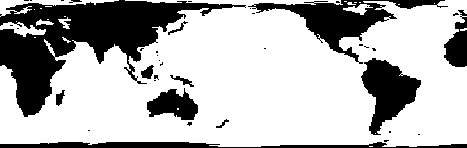# areamat

Surface area covered by nonzero values in binary data grid

## Syntax

```A = areamat(BW,R) A = areamat(BW,R,ellipsoid) [A, cellarea] = areamat(...) ```

## Description

`A = areamat(BW,R)` returns the surface area covered by the elements of the binary regular data grid `BW`, which contain the value 1 (`true`). `BW` can be the result of a logical expression such as `BW = (topo60c > 0)`. Specify `R` as a `GeographicCellsReference` object. The `RasterSize` property of `R` must be consistent with `size(BW)`.

The output `A` expresses surface area as a fraction of the surface area of the unit sphere (4*pi), so the result ranges from 0 to 1.

`A = areamat(BW,R,ellipsoid)` calculates the surface area on the ellipsoid or sphere defined by the input `ellipsoid`, which can be a `referenceSphere`, `referenceEllipsoid`, or `oblateSpheroid` object, or a vector of the form ```[semimajor_axis eccentricity]```. The units of the output, `A`, are the square of the length units in which the semimajor axis is provided. For example, if `ellipsoid` is replaced with `wgs84Ellipsoid('kilometers')`, then `A` is in square kilometers. If you do not specify `ellipsoid` and `R` has a non-empty `GeographicCRS` property, then `areamat` uses the ellipsoid contained in the `Spheroid` property of the `geocrs` object in the `GeographicCRS` property of `R`.

`[A, cellarea] = areamat(...)` returns a vector, `cellarea`, describing the area covered by the data cells in `BW`. Because all the cells in a given row are exactly the same size, only one value is needed per row. Therefore `cellarea` has size `M-by-1`, where `M = size(BW,1)` is the number of rows in `BW`.

## Examples

collapse all

Find the surface area in normalized units of the part of Earth's terrain that is above sea level.

First, load elevation raster data and a geographic cells reference object. The raster contains terrain heights relative to mean sea level. Then, create a logical array representing the terrain above sea level.

```load topo60c topoASL = topo60c > 0;```

Find the surface area in normalized units of the elements of the array that contain `true`.

`areamat(topoASL,topo60cR)`
```ans = 0.2890 ```

The result means 28.9% of Earth's terrain is above sea level.

Find the surface area in kilometers of the part of Earth's terrain that is above sea level.

First, load elevation raster data and a geographic cells reference object. The raster contains terrain heights relative to mean sea level. Then, create a reference sphere for Earth and specify its units as kilometers.

```load topo60c s = referenceSphere('earth','km');```

Create a logical array representing the terrain above sea level.

`topoASL = topo60c > 0;`

Find the surface area in kilometers of the elements of the array that contain `true`.

`areamat(topoASL,topo60cR,s)`
```ans = 1.4739e+08 ```

The result means that approximately 147 million square kilometers of Earth's terrain is above sea level.

## Tips

Given a regular data grid that is a logical 0-1 matrix, the `areamat` function returns the area corresponding to the true, or 1, elements. The input data grid can be a logical statement, such as `(topo60c > 0)`, which is 1 everywhere that `topo60c` is greater than 0 meters, and 0 everywhere else. This is an illustration of that matrix:This calculation is based on the `areaquad` function and is therefore limited only by the granularity of the cellular data.

## Version History

Introduced before R2006a

expand all